# Finite-Dose Models of Transient Exposures and Volatile Formulation Components

Fig. 8.1
Schematic representation of flux and cumulative absorption profiles for a finite-dose application to human skin (© Williams (2003), used with permission)

Designing in vitro experiments that accurately reflect this process may be difficult. Application of materials to the skin surface in volatile solvents may result in damage to the skin barrier, usually by delipidisation. Such damage is normally minimised by the use of volatile solvents which are removed rapidly from the skin surface. This will also result in a thin film of solid solute being deposited on the skin surface. The use of a volatile liquid solvent to achieve this results in greater accuracy in dosing as it removes issues associated with gravimetric dosing, being easier to administer to small diffusion cells, and also allows the dose to be more evenly applied to the skin surface (Williams 2003). It follows from such a discussion that the development of quantitative models describing such processes will be equally challenging.

Infinite-dose profiles usually report the steady-state flux of the absorption process, or its derivative, kp, the permeability coefficient. In the case of finite-dose experiments, the maximum flux (J max) and the time to reach maximum flux (T max) are often reported. Crank (1975) showed that J max may be defined as:(8.1)
where

D

is the apparent diffusion coefficient;

C 0

is the concentration of the permeant in the first layer of the stratum corneum, which is maximal when solid deposited drug is in contact with the skin surface;

h

is the thickness of the stratum corneum;

δ

is the thickness of the finite-dose layer on the skin surface.

T max may be represented by the expression:(8.2)
where the terms used in Eq. 8.2 are as defined for Eq. 8.1.

As a finite dose, δ, is significantly smaller than the thickness of the stratum corneum, Eq. 8.2 is often simplified to:(8.3)

These equations allow the estimation of the apparent diffusion coefficient, although any such value should be calculated in the context of the limitations of the finite-dose model described above.

Franz et al. (1993) illustrated the differences in finite- and infinite-dose systems by comparing the permeation of N-nitrosodiethanolamine (NDELA) through human skin in vitro. Their results, for a range of cosmetic vehicles, are summarised in Table 8.1. They show that for an infinite dose of NDELA (500 μg/cm2 at 1 μg/μl), the total dose absorbed was formulation dependant. There was also evidence that the rate of absorption increased with time which the authors claimed suggested skin barrier damage. Three finite doses were applied (0.6, 2.0 and 6.0 μg/cm2, applied as 10 μl of 0.06, 0.2 and 0.6 μg/μl, respectively) to the skin, and the percentage absorption was found to be largely concentration independent as the total dose absorbed (as μg/cm2) was directly proportional to the concentration of NDELA in the vehicle. As with infinite-dose studies, the total absorbed at the end of the 48-h experiment was formulation dependent. Table 8.1 shows a comparison of the infinite- and finite-dose experiments, with absorption presented as a percentage of the applied dose. For all formulations, it is apparent that the finite-dose experiments result in greater percentage permeation.

Table 8.1
Percutaneous penetration of N-nitrosodiethanolamine (NDELA) through human skin in vitro for a range of cosmetic formulations in both finite- and infinite-dose systems

Infinite dose (500 μl/cm2)

Finite dose

10 μl/cm2

10 μl/cm2

10 μl/cm2

Total dose (μg/cm2)

500

0.6

2.0

6.0

Concentration (μg/μl)

1.0

0.06

0.20

0.6

IPM

24.9 + 5.2

41.8 + 10.9

65.2 + 9.5

61.9 + 13.9

Shampoo

35.4 + 7.6

42.7 + 15.1

42.9 + 13.7

46.4 + 12.4

Sunscreen

3.9 + 1.6

37.3 + 12.3

35.3 + 8.3

41.4 + 14.5

Modified from Franz et al. (1993)

As such experiments provide experimental data for modelling studies, there has emerged a body of research that has focused on the use of finite-dose models in characterising skin permeation in a range of systems (for pharmaceutical, bulk industrial, cosmetic and risk assessment/toxicity purposes). The rest of this chapter will discuss such models.

## Modelling Finite-Dose Experiments

Finite-dose systems are usually associated with transient or unoccluded applications of small amounts to the skin surface. This may occur in a range of contexts, from pesticide application to crops, to industrial exposures associated with volatile solvents or the topical application of unoccluded medicines such as creams or ointments. A number of studies have examined dermal absorption under such circumstances and have provided a platform from which models have been subsequently developed. For example, a number of studies on the dermal absorption of aviation fuel have indicated that absorption depends on the type of fuel and its specific components, with some materials resulting in different peak absorptions; for example, Riviere et al. (1999) determined that naphthalene had a peak flux 1 h following a transient exposure whereas dodecane and hexadecane had prolonged, but lower, fluxes. A subsequent study found that there are no significant differences in the ability of four different fuels to influence absorption (Baynes et al. 2000).

Other topically applied volatile chemicals have also been examined. Riviere et al. (2000) examined the absorption of chloropentafluorobenzene and dichlorobenzene, suggesting that dosing methodology is a major factor in the degree of subsequent dermal absorption and that methodological factors could influence measurement of such uptake. As “real-world” formulations applied to the skin are seldom simple, several studies have explored the effect of solvent mixtures on skin absorption. Baynes et al. (2001) investigated mixture effects on the dermal absorption of aviation fuel components. They found, after a series of experiments which examined systematic variations in the composition of performance additives, that such changes significantly altered the dermal disposition of aromatic and aliphatic fuel components within the skin. They also found that the products of two-factor interactions could not be predicted from two, single-factor exposures. Riviere et al. (2001) examined the influence of mixture effects on the dermal absorption of pentachlorophenol and concluded that dermal absorption estimates from single chemical exposures do not reflect the dermal absorption measured following application of chemical mixtures. This theme is discussed in the following section in more detail.

Frasch and Barbero (2008) considered several examples of dermal absorption following transient exposure, using infinite-dose experiments (permeability and lag time) to predict the accumulation of chemicals in the skin following transient exposure and by applying Laplace domain solutions to their theoretical framework. They considered the skin as a homogenous membrane which is free of chemicals at the beginning of the experiment, after which time a chemical of known concentration (C 1) is applied for a finite time (T 1), after which it is completely removed. In using the one-dimensional diffusion equation: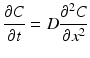(8.4)
where

D

is diffusivity;

t

is time;

x

is position.

Frasch and Barbero then defined the flux as:(8.5)

Equation 8.5 is then integrated with respect to t, which gives the total mass accumulated per unit area after time t:(8.6)
They then considered a number of exposure conditions. These included the situation of zero concentration at the upper surface (the outer surface of the skin). In this scenario, the concentration at the surface of the skin is maintained at zero, which reflects an infinite, well-stirred reservoir on the skin surface for a highly volatile compound. In this case, the chemical diffuses upwards through the skin and immediately evaporates when it reaches the skin surface, being carried into the surrounding environment. In this case, the total mass accumulation is defined as:(8.7)
where

m

is the total mass accumulation as time approaches infinity;

k p = K mv.D/h

the permeability coefficient, where K mv is the membrane–vehicle partition coefficient;

A i

is the area of skin exposed to the chemical;

C i and T i

relate to multiple intermittent exposures of the skin of concentration C i for duration T i .

They also considered the scenario where zero flux was observed at the upper surface of the skin. Following the exposure period, the upper skin surface presents an impermeable barrier to diffusion and represents, in this model, the potential permeation of a non-volatile compound where absorption into the skin is energetically preferred to evaporation into the surrounding environment. In this case, the total mass accumulation as time approaches infinity is represented by the sum of the amount in the membrane at time T i :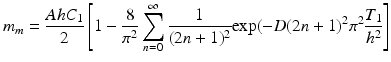(8.8)
where

T 1

is the amount that has passed through the membrane at time t;

h

is the thickness of the membrane.

The sum of the mass, rewritten in terms of permeability coefficient and lag time, is: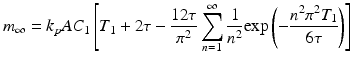(8.9)
where= h 2/(6D), the membrane lag time.

For long exposure times, the exponential component of Eq. 8.9 becomes negligible, allowing the approximation shown in Eq. 8.10 to be used as: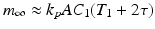(8.10)

And, for very long exposure times, such as when T 1 is significantly greater than 2, Frasch and Barbero suggested that Eq. 8.10 could be simplified further to: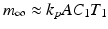(8.11)

Comparison with Eq. 8.7 suggests that the total mass accumulation is the same in both scenarios and that a similar situation may occur when the lag time is very small.

They then applied their theoretical considerations to the in vitro permeation of diethylphthalate applied to hairless guinea pigskin and to silicone membranes as transient exposures. In general, they found excellent correlations between their theoretical framework and the experimental results, with r 2 values ranging from 0.97 to 0.999. Even though the discrepancies between theoretical estimates and practical observations are very minor, the authors considered the use of silicone membranes to explore issues with skin heterogeneity, suggesting that transient dose experiments supported to a degree the homogenous membrane theory proposed in their theoretical framework along with other factors, such as the finite time required to wash membranes which, while kept as brief as possible, may still have resulted in some diffusion during the process, or the potential for their model penetrant (diethylphthalate) to bind to skin components.

A two-stage kinetic analysis was adopted by Saiyasombati and Kasting (2003) to characterise fragrance evaporation and absorption following topical application to the skin. Their model considered low doses—typically associated with the topical application of fragrances from a range of vehicles—and used a previously published data set containing evaporation rates of topically applied volatile chemicals. Evaporation rates from formulations containing a musk fixative (a commonly used ingredient to retard evaporation of substances from the skin surface and increase the life of a fragrance on the skin) followed single exponential decays that could be described by a single-compartment model. In contrast, the same system without the musk fixative resulted in two-compartment biexponential decay. None of the models presented accounted for the ingredient interactions that lead to the observed differences, which led the authors to suggest the adoption of a diffusion/evaporation model in subsequent studies. Nevertheless, their compartmental kinetic models yielded reasonable correlations with the total evaporated fraction of each component in the formulations evaluated and implied a relationship, in the absence of skin binding or chemical reactivity, between molecular properties of an ingredient and the fraction of the dose absorbed. They concluded that the two-compartment model, where evaporation from the vehicle and skin was both considered, provided the most useful model in quantifying the duration of the fragrances on the skin.

Miller et al. (2005) investigated the effect of dose and airflow dependence on the disposition of benzyl alcohol on the skin. They showed that the dose absorbed after 24 h increased with the amount applied to the skin for nine different doses. Less than 4 % of the radiolabelled active was retained in the tissue after this time and the rest was assumed to have evaporated. Analysis of their data showed that the increase in benzyl alcohol absorption was consistent with an increase in diffusivity in the stratum corneum, as the concentration increased. Their variable diffusivity model (Eq. 8.12) was able to estimate the observations to within 4.2 % of dose: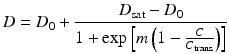(8.12)
where

D

is the diffusivity of the permeant in the membrane,

D 0 and D sat

reflect the concentration-dependent diffusion coefficient which varied from approximately D 0 at low concentrations to D sat at the saturation concentration, C sat,

C = C(x)

the local concentration of permeant in the membrane.

They concluded that the experimentally measured dose- and airflow-dependant absorption and evaporation of benzyl alcohol in vitro fit well to their proposed model and were consistent with a finite-dose diffusion model describing evaporative loss from the skin surface and concentration-dependant diffusion from the skin surface. In addition, while Miller et al. discussed the relevance and validity of their predictive model, they also highlighted its application to a limited number of chemicals.